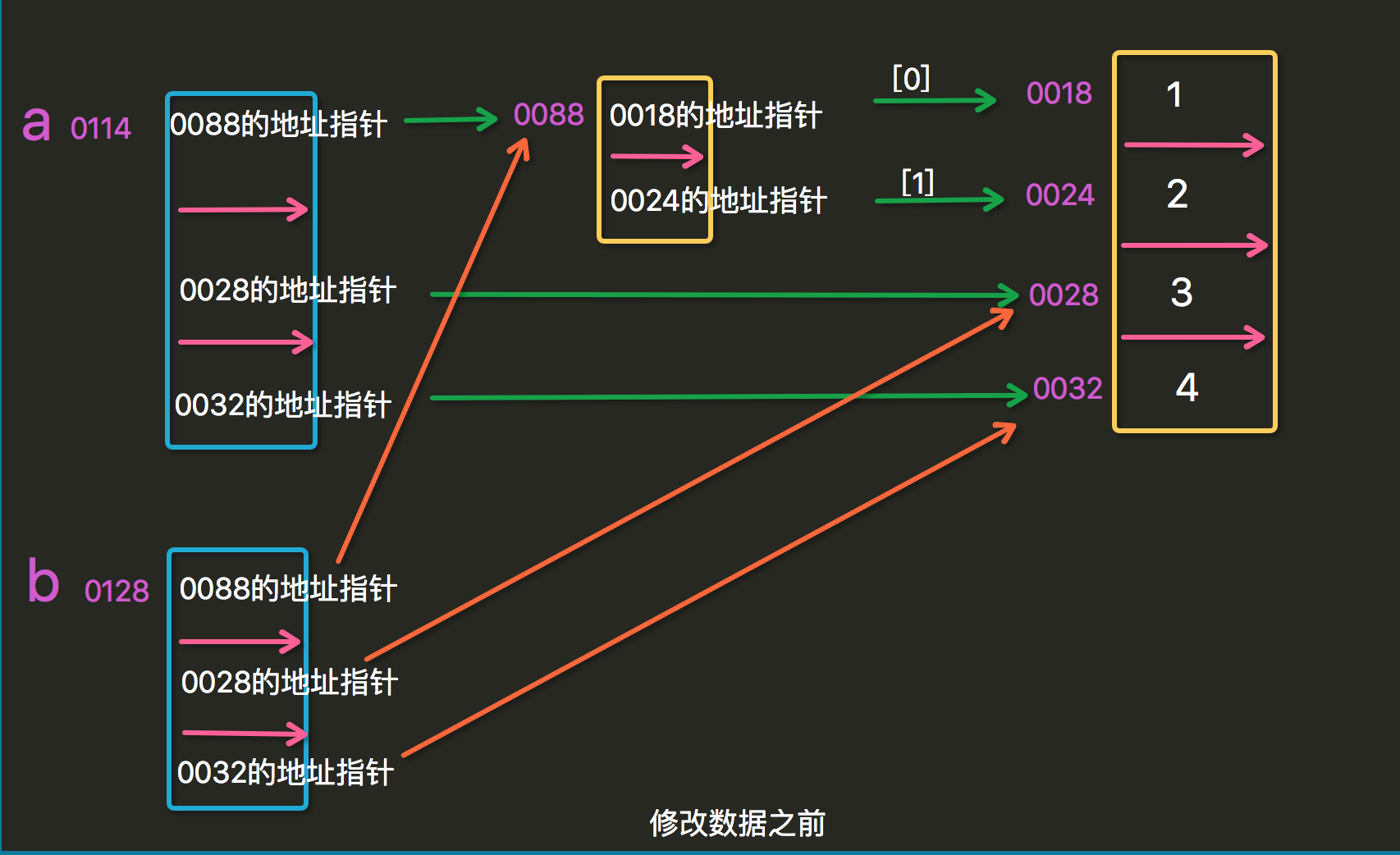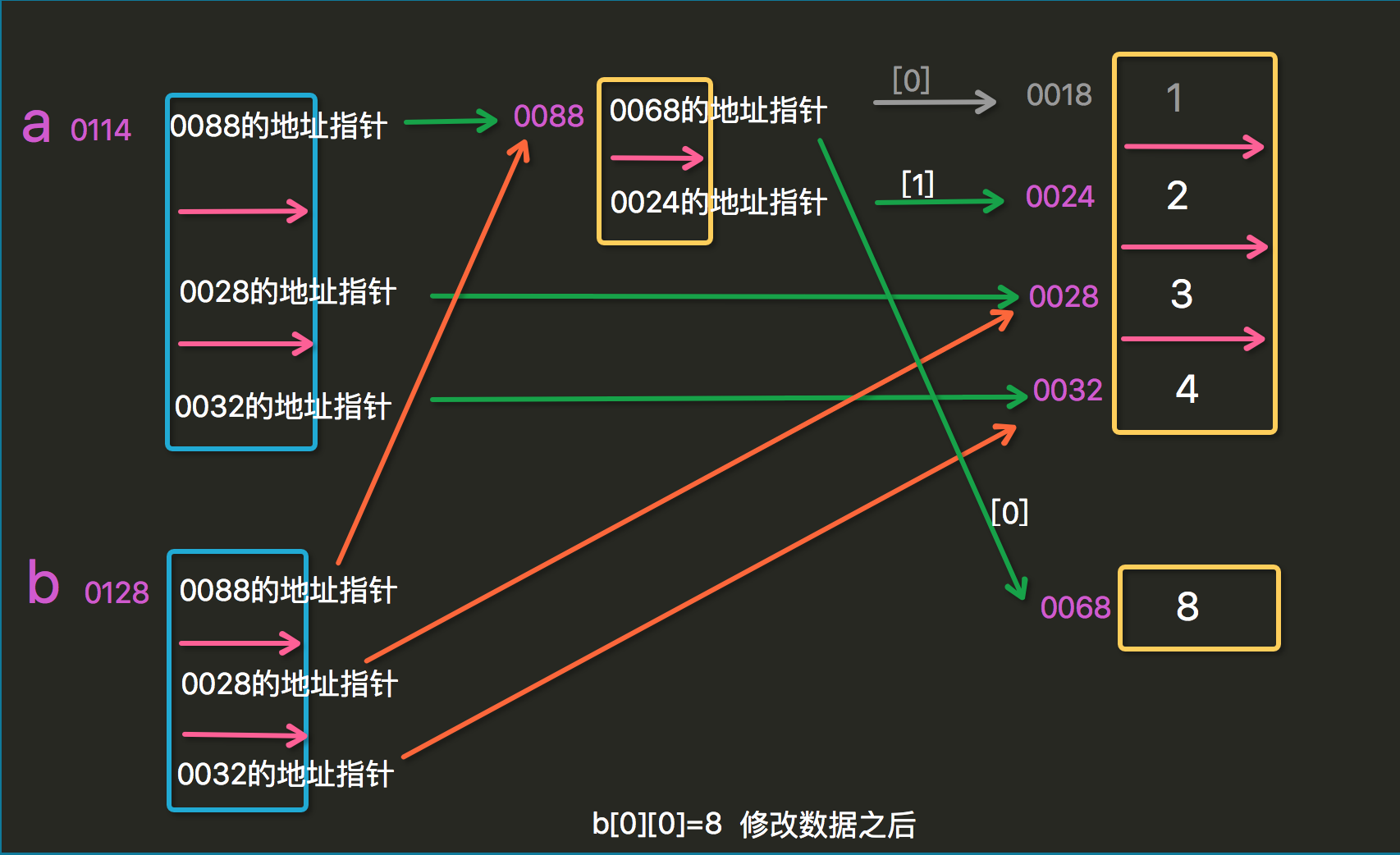# Python学习(006)-深浅拷贝及集合

深浅拷贝1 import copy
2
3 husband=['xiaoxin',123,[200000,100000]]
4
5 wife=husband.copy()   #浅拷贝
6 wife='xiaohong'
7 wife='456'
8
9 xiaosan=copy.deepcopy(husband)  #深拷贝
10 xiaosan='xiaoyu'
11 xiaosan='666'
12
13 wife -=2000
14 xiaosan -=1999
15
16 print(husband,wife,xiaosan)
View Code

['xiaoxin', 123, [200000, 98000]] ['xiaohong', '456', [200000, 98000]] ['xiaoyu', '666', [200000, 98001]]


浅拷贝，只会拷贝第一层修改数据后：集合

• 去重，把一个列表变成集合，就自动去重了
• 关系测试，测试两组数据之前的交集、差集、并集等关系s=set('Nicknick')
s1=['nick','xiaoli','xiaozhang','nick']
print(s)   #{'c', 'i', 'N', 'k'}
s1=set(s1)
print(s1)  #{'xiaoli', 'nick', 'xiaozhang'}
View Code

s = set([3,5,9,10])      #创建一个数值集合

t = set("Hello")         #创建一个唯一字符的集合

a = t | s          # t 和 s的并集

b = t & s          # t 和 s的交集

c = t – s          # 求差集（项在t中，但不在s中）

d = t ^ s          # 对称差集（项在t或s中，但不会同时出现在二者中）

s.update([10,37,42])  # 在s中添加多项

t.remove('H')

len(s)
set 的长度

x in s

x not in s

s.issubset(t)
s <= t

s.issuperset(t)
s >= t

s.union(t)
s | t

s.intersection(t)
s & t

s.difference(t)
s - t

s.symmetric_difference(t)
s ^ t

s.copy()

a=set([1,2,3,4,5])
b=set([4,5,6,7,8])

#交集
print(a.intersection(b))   #{4, 5}
print(a&b)

#并集
print(a.union(b))    #{1, 2, 3, 4, 5, 6, 7, 8}
print(a|b)

#差集
print(a.difference(b))    #{1, 2, 3}
print(a-b)
print(b.difference(a))    #{8, 6, 7}
print(b-a)

#对称差集  反向交集
print(a.symmetric_difference(b))      #{1, 2, 3, 6, 7, 8}
print(a^b)

#父集  超级
print(a.issuperset(b))
print(a>b)                 #False

#子集
print(a.issubset(b))
print(a<b)              #False

posted @ 2018-10-10 14:29  Nick1994  阅读(79)  评论(0编辑  收藏